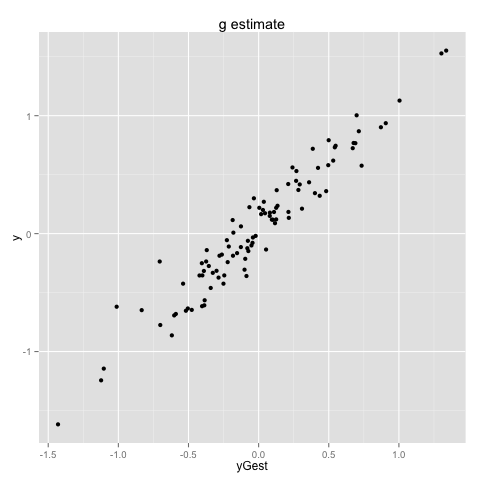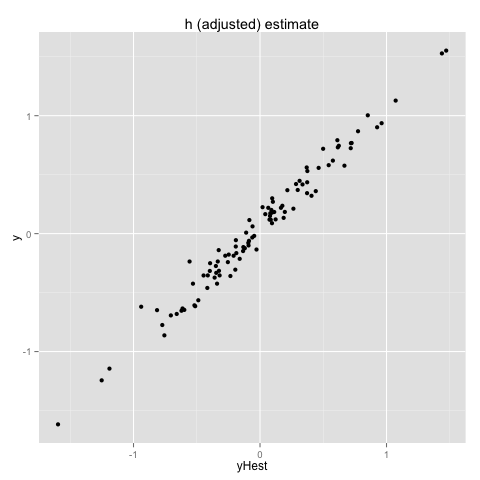iPython notebook source here

Example of using methods from the orthogonal Procrustes problem ( Schonemann, P.H. (1966), A generalized solution of the orthogonal Procrustes problem, Psychometrika 31: 1–10 ) to try and solve for simple x concept drift. For notes on deriving the Procrustes solution see the application and the derivation.

The ideas is we have a random process generating y_i ~ b . z_i + e_i where y_i is the scalar outcome, z_i is a vector, b is a vector and e_i is the scalar error term. We further assume that z_i is in fact unobserved and we actually observe pairs (y_i, x_i) where x_i ~ L_1 z_i where L_1 is an unobserved linear operator. The drift idea is after observing n1 observation pairs we switch to observing only q_i where q_i ~ L_2 z_i (L_2 a new unobserved linear operator) and would like to estimate the now unobserved y_i.

We know y_i ~ b L_1^{-1} x_i and can build an estimate g ~ b L_1^{-1} from the initial data. After the switch over we know the unobserved y_i are ~ b L_2^{-1} x_i so we want an h ~ L_2^{-1}. h ~ g L_1 L_2^{-1} would work. So we need an estimate of L_1 L_2^{-1} from our observations of only the x_i and the q_i.

For this we appeal to the orthogonal Procrustes problem to find an operator r that sends the observed inertial ellipsoid sum_{i=1...n2} q_i transpose(q_i)/n2 to sum_{i=1...n1} x_i transpose(x_i)/n1 (we call the routine find correction). We then use the estimate y_i ~ g . r q_i.

In :
%load_ext rpy2.ipython

In :
# import packages
import numpy
import math
import pandas
import sklearn.linear_model

In :
%%R
library(ggplot2)
library(reshape2)

In :
# define functions

# e1, e2 positive semi-definite square numpy matrices (of same shape)
# each one represents an ellipsoid by the quadratic form over vectors
# as numpy.transpose(x) * ei * x
def findCorrection(e1,e2):
# make sure we are working with matrices, so * is matrix muliplication, not element-wise muliplication
e1 = numpy.matrix(e1)
e2 = numpy.matrix(e2)
# first find principal axes of ellipsoids e1, e2
u1,s1,v1 = numpy.linalg.svd(e1)
l1 = u1*numpy.matrix(numpy.diag([math.sqrt(si) for si in s1]))*v1
l1inv = numpy.transpose(v1)* \
numpy.matrix(numpy.diag([math.sqrt(1.0/si) if si>1.0e-5 else 0.0 for si in s1]))* \
numpy.transpose(u1)
u2,s2,v2 = numpy.linalg.svd(e2)
l2 = u2*numpy.matrix(numpy.diag([math.sqrt(si) for si in s2]))*v2
l2inv = numpy.transpose(v2)* \
numpy.matrix(numpy.diag([math.sqrt(1.0/si) if si>1.0e-5 else 0.0 for si in s2]))* \
numpy.transpose(u2)
# Find linear transform r from e2 frame to e1 frame.
# We want r * l2 = l1 and r near the identity matrix.
# Write r = l1 * o * l2inv * x, where o is
# an orthonormal matrix chosen so l1 * o * l2inv is
# near the identity matrix.  We know how to minimize |A o - B|F
# for orthognal o (||F Froebenious norm).  This is not the same
# as minimizing |A o B - I|F, but we will use the method we have
# available.
# So we are deliberatly sloppy and will choose to interpret
# "near the identity" as |o - l1^{-1} l2|F small
# so we can solve by treating as an orthogonal Procrustes problem.
u3,s3,v3 = numpy.linalg.svd(l1inv*l2)
r = l1*u3*v3*l2inv
return r

# frame a Pandas dataframe
# varNames a list of strings
# get inertial ellipsoid from a pandas data frame for set of columns
def getEllipsoid(frame,varNames):
mat = numpy.matrix(frame[varNames].values)
return numpy.transpose(mat)*mat/(1.0*mat.shape)

In :
# simple example

e1 = [[1.1,0.0],[0.0,0.9]]
e2 = [[0.9,0.0],[0.0,1.1]]
r = findCorrection(e1,e2)
print 'r',type(r),r.shape,r

print 'e1 est from e2',r.transpose()*e2*r

r <class 'numpy.matrixlib.defmatrix.matrix'> (2, 2) [[ 1.1055416   0.        ]
[ 0.          0.90453403]]
e1 est from e2 [[ 1.1  0. ]
[ 0.   0.9]]


In :
# build data driven example

numpy.random.seed(29232295)
dim = 3
n1 = 100
n2 = 100
sameRows = False
if sameRows:
n2 = n1
varNames = [ 'x' + str(i) for i in range(dim) ]
colNames = varNames + ['y']
print(colNames)
L1 = numpy.matrix(numpy.identity(dim) + 0.1*numpy.random.randn(dim,dim))
L2 = numpy.matrix(numpy.identity(dim) + 0.1*numpy.random.randn(dim,dim))
#L1 = numpy.matrix(numpy.identity(dim))
#L2 = numpy.matrix(numpy.identity(dim))
b = numpy.matrix(numpy.random.randn(1,dim))
noiseMagnitude = 0.1

print "b",type(b),b.shape,b
print "L1",type(L1),L1.shape,L1
print "L2",type(L2),L2.shape,L2

copies = []

# build training data
trainFrame = pandas.DataFrame(columns = colNames)
for i in range(n1):
zi = numpy.matrix(numpy.random.randn(dim,1))
if sameRows:
copies.append(zi)
yi = b.dot(zi)[0,0] + noiseMagnitude*numpy.random.rand()
xi = L1*zi
newRow = [ xi[j,0] for j in range(dim) ]
newRow.append(yi)
trainFrame.loc[i] = newRow

# build applied data
appFrame = pandas.DataFrame(columns = colNames)
for i in range(n2):
if sameRows:
zi = copies[i]
else:
zi = numpy.matrix(numpy.random.randn(dim,1))
yi = b.dot(zi)[0,0] + noiseMagnitude*numpy.random.rand()
qi = L2*zi
newRow = [ qi[j,0] for j in range(dim) ]
newRow.append(yi)
appFrame.loc[i] = newRow

['x0', 'x1', 'x2', 'y']
b <class 'numpy.matrixlib.defmatrix.matrix'> (1, 3) [[-0.2468905   0.11248646 -0.45424536]]
L1 <class 'numpy.matrixlib.defmatrix.matrix'> (3, 3) [[ 0.98361632  0.07897896  0.18438749]
[ 0.0422153   0.9860273   0.09071934]
[-0.03331292  0.15028965  1.0828952 ]]
L2 <class 'numpy.matrixlib.defmatrix.matrix'> (3, 3) [[ 0.9751936  -0.16789224 -0.00915064]
[ 0.05367467  0.93461914  0.11486113]
[-0.15742225  0.01337319  1.04831824]]


In :
# fit the model
#g = numpy.matrix(numpy.linalg.lstsq(trainFrame[varNames].values,trainFrame['y']))
clf = sklearn.linear_model.Ridge(copy_X=True, fit_intercept=False, normalize=False, alpha=0.1)
g = numpy.matrix(clf.fit(trainFrame[varNames].values,trainFrame['y']).coef_)
print 'g estimate',g
print 'unobserved train b estimate',g*L1  # L1 would not be known to an experimentor
print 'unosberved b',b

e1 = getEllipsoid(trainFrame,varNames)
print 'e1',type(e1),e1.shape,e1

e2 = getEllipsoid(appFrame,varNames)
print 'e2',type(e2),e2.shape,e2

r = findCorrection(e1,e2)
print 'estimatedCorrection',type(r),r.shape,r
print 'estimate Froebenious norm from identity',numpy.linalg.norm(r-numpy.identity(dim),'fro')

print 'e1 est from e2 correction',r.transpose()*e2*r

xformHidden = (L1*numpy.linalg.inv(L2)).transpose()
print 'xformHidden',type(xformHidden),xformHidden.shape,xformHidden
print 'xform Froebenious norm from identity',numpy.linalg.norm(xformHidden-numpy.identity(dim),'fro')
print 'e1 est from e2 xformHidden',xformHidden.transpose()*e2*xformHidden
print 'unobserved transformed estimate',g*xformHidden.transpose()

#hHidden = numpy.matrix(numpy.linalg.lstsq(appFrame[varNames].values,appFrame['y']))
clfH = sklearn.linear_model.Ridge(copy_X=True, fit_intercept=False, normalize=False, alpha=0.1)
hHidden = numpy.matrix(clfH.fit(appFrame[varNames].values,appFrame['y']).coef_)

# adjust coefficients
print 'hHidden',hHidden
print 'unobserved train b estimate',hHidden*L2  # L2,hHidden would not be known to an experimentor

hEst = g*r.transpose()
print 'hEst',type(hEst),hEst.shape,hEst

g estimate [[-0.2675406   0.19573146 -0.39487157]]
unobserved train b estimate [[-0.24174012  0.11252138 -0.45917904]]
unosberved b [[-0.2468905   0.11248646 -0.45424536]]
e1 <class 'numpy.matrixlib.defmatrix.matrix'> (3, 3) [[ 1.09202485  0.18558626  0.2631439 ]
[ 0.18558626  0.91587241  0.25676302]
[ 0.2631439   0.25676302  1.11305653]]
e2 <class 'numpy.matrixlib.defmatrix.matrix'> (3, 3) [[ 1.0619409  -0.17264232 -0.16900112]
[-0.17264232  0.98282496  0.31181581]
[-0.16900112  0.31181581  1.23567532]]
estimatedCorrection <class 'numpy.matrixlib.defmatrix.matrix'> (3, 3) [[  1.03354972e+00   1.45370099e-01   1.47631097e-01]
[  1.67885970e-01   9.82482345e-01  -1.06845734e-02]
[  2.02640207e-01   9.94363280e-04   9.58209718e-01]]
estimate Froebenious norm from identity 0.339809045087
e1 est from e2 correction [[ 1.10334624  0.19937212  0.27489248]
[ 0.19937212  0.92238125  0.25885977]
[ 0.27489248  0.25885977  1.10415758]]
xformHidden <class 'numpy.matrixlib.defmatrix.matrix'> (3, 3) [[ 1.01916763 -0.0192741   0.12054787]
[ 0.26535617  1.05195543  0.16792552]
[ 0.15571075 -0.02888991  1.01563641]]
xform Froebenious norm from identity 0.376706628943
e1 est from e2 xformHidden [[ 1.08095407  0.117888    0.24869385]
[ 0.117888    1.07688986  0.44908736]
[ 0.24869385  0.44908736  1.37575606]]
unobserved transformed estimate [[-0.32404219  0.06859821 -0.44835955]]
hHidden [[-0.33033421  0.06523913 -0.44242063]]
unobserved train b estimate [[-0.24899127  0.11051771 -0.4532814 ]]
hEst <class 'numpy.matrixlib.defmatrix.matrix'> (1, 3) [[-0.30635833  0.15160543 -0.43238963]]


In :
# make predictions using adjusted coefficients
# go through all of the trouble of getting in and out of matrix notation
def applyEst(frame,coefs):
return numpy.squeeze(numpy.asarray(numpy.matrix(frame[varNames].values)*(coefs.transpose())))

appFrame['yGest'] = pandas.Series(applyEst(appFrame,g),index=appFrame.index)
appFrame['yHest'] = pandas.Series(applyEst(appFrame,hEst),index=appFrame.index)

print 'g estimate square error',sum([(appFrame['yGest'][i]-appFrame['y'][i])**2 for i in range(n2)])
print 'h estimate square error',sum([(appFrame['yHest'][i]-appFrame['y'][i])**2 for i in range(n2)])

g estimate square error 2.67619425173
h estimate square error 1.07708434887


In :
# make same predictions using adjusted coordinates

yGest = clf.predict(appFrame[varNames].values)
yHest = clf.predict(appFrame[varNames].values*r)

print 'g estimate square error',sum([(yGest[i]-appFrame['y'][i])**2 for i in range(n2)])
print 'h estimate square error',sum([(yHest[i]-appFrame['y'][i])**2 for i in range(n2)])

g estimate square error 2.67619425173
h estimate square error 1.07708434887


In :
%%R -i appFrame
appFrame <- as.data.frame(appFrame)
print(summary(appFrame))
print(paste('RMS g',mean((appFrame$yGest-appFrame$y)^2)))
print(paste('RMS h',mean((appFrame$yHest-appFrame$y)^2)))
print(ggplot(data=appFrame) + geom_point(aes(x=yGest,y=y)) + ggtitle("g estimate"))
print(ggplot(data=appFrame) + geom_point(aes(x=yHest,y=y)) + ggtitle("h (adjusted) estimate"))

       x0                 x1                 x2                  y
Min.   :-2.65631   Min.   :-3.34399   Min.   :-2.538284   Min.   :-1.61731
1st Qu.:-0.65545   1st Qu.:-0.68815   1st Qu.:-0.633643   1st Qu.:-0.31558
Median :-0.01663   Median : 0.08632   Median : 0.006595   Median : 0.03485
Mean   :-0.02383   Mean   :-0.03239   Mean   : 0.026068   Mean   : 0.04075
3rd Qu.: 0.68427   3rd Qu.: 0.56412   3rd Qu.: 0.836432   3rd Qu.: 0.36875
Max.   : 2.05161   Max.   : 2.38584   Max.   : 2.377135   Max.   : 1.55191
yGest              yHest
Min.   :-1.43014   Min.   :-1.596740
1st Qu.:-0.33046   1st Qu.:-0.341212
Median :-0.04218   Median :-0.052606
Mean   :-0.01026   Mean   :-0.008882
3rd Qu.: 0.27287   3rd Qu.: 0.318612
Max.   : 1.33709   Max.   : 1.471736
 "RMS g 0.0267619425172501"
 "RMS h 0.010770843488707"In :